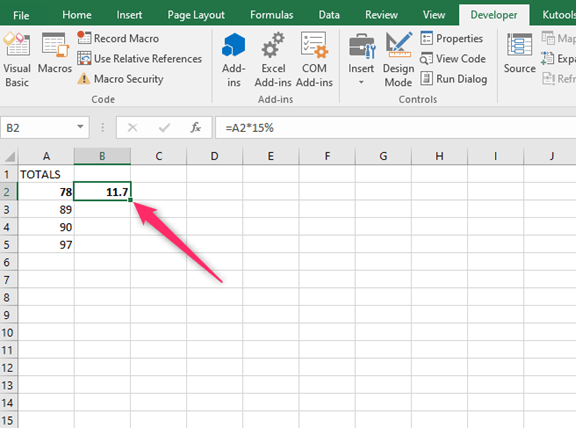# The Shortcut to Apply a Formula to an Entire Column in Excel

Since formulas are the most used elements in Excel, they are not just used in one or a couple of cells. Formulas are applied in an entire column or a large range of cells in the column. There are several ways that Excel gives you to apply a formula to an entire column in Microsoft Excel. However, the keyboard shortcut CTRL+ D is the shortest method.

## Using Keyboard Shortcut

Use the easy steps below as you apply the keyboard shortcut method;

1. Enter the formula =A2*15% in the B2 cell.2. Select all the "cells" that you need to apply the formula3. Press CTRL+ D keys or drag.Double- Clicking on the AutoFill Handle

It is one of the simplest ways to use the mouse double-clicking method. The formula to use may be =B2*15%. Use the following simple steps to apply the formula.

1. Enter the formula =A2*15% in the cell B22. Select the "cells" you need to apply this action3. A small green square appears at the bottom-right part of your selection. Position the cursor above the green square. This action changes the cursor to a plus sign.4. Double click the left mouse key

The entire column is automatically filled up to the cells adjacent to your column. It is important to note that there should be no data in the adjacent column or blank cells so that this method can work.

## Using the Drag and Autofill Handle

This method can be applied when you have a small dataset; hence you can manually drag the fill handle to apply the formula.

1. Enter =A2*15% in the B2 cell

2. Select the "cells" you need to apply this action, and a small green box appears at the bottom-right of the selection you have just made3. Position the cursor above the small green square; it changes to a plus sign

4. Holding the left key of the mouse, drag it to the cell that you want to apply the formula, then release the mouse

## By using the Fill Down Option

You first have to select the cells you want to have the formula applied. Use the simple steps below;

1. Enter the formula =A2*15%

2. Make a selection of all the cells that you want the formula to be applied to3. Go to the Home Tab

4. Select the Fill icon in the editing group5. Go to Fill DownThe formula is taken from C2 and then filled in all selected cells.